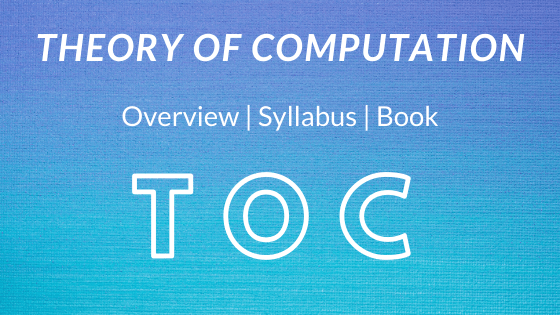# Theory of Computation (TOC) Overview## Introduction of Theory of Computation (TOC)

Automata theory (also identified as Theory Of Computation) is a theoretical department of Computer Science and Mathematics, which mostly concerned with the good judgment of computation with respect to straight forward machines, generally known as automata.

The key motivation in the back of constructing the Automata idea was to describe and analyze the dynamic behavior of discrete systems.

The word “Automata” is obtained from the Greek word “αὐτόματα” which signifies “self-acting”. which is firmly identified with “Automation”.

An automaton is used for performing some functions without direct participation of man.

Example = automatic machine tools, automatic packing machines, and automatic photo printing machines, etc.

## Types Of Automata

1. Automation without a memory: output depends only on the input

2. Automaton with a finite memory: output depends not only depends on the input as well as output too

3. Moore machine: the output depends only on the states of the machine

4. Mealy machine.: the output depends on the state as well as on the input at any instant of time

DFA NDFA
• Deterministic Finite Automata (DFA)
• Non-deterministic Finite Automata (NFA)
• Null (or ε) move is not allowed
• Null (or ε) move is allowed
• Q: set of all states.
• Q: set of all states.
• ∑: set of input symbols.
( Symbols which machine takes as input)
• ∑: set of input symbols.
( Symbols which machine takes as input)
• q: Initial state
• F: set of final state.
• δ Transition Function
δ: Q X Σ → Q
• q: Initial state
• F: set of final state.
• NFA Transition Function:
δ: Q X ( Σ U ε )2Q

## Some Important terminology used in Theory of Computation (TOC)

Here I am writing only Important Terminology of TOC, for Mathematical Terminology you have to visit Mathematical Notions And Terminology post also.

• Symbols: Any letter, alphabet, or any picture.
E.g.: a, b, c, 0, 1, 2 ……
• Alphabets: Finite set of symbols.
It is denoted by Σ
Σ is a collection of symbols.
E.g.: {a, b}, {0, 1, 2}
• String: Sequence of symbols.
E.g.: a, b, 0, 1, aa, bb, ab, 01, …
• Language: A Language is a collection of string.
Set of strings.
A language that is formed over Σ, It may be finite and infinite.
E.g.: Σ={0,1}

• Automata: For solving the infinite language we used automata
It is a mathematical model or machine
whether the string is a part of language or not
• Power of  Σ:
Σ = {a,b} then
Σ° =
Set of all string over Σ of length 0, {ε}
Σ¹ =
Set of all strings over Σ of length 1, {a,b}
Σ² =
Set of all strings over Σ of length 2, {aa, ab, bb, ba}
• Cardinality: Number of elements in a set
Σ° Cardinality is 1
Σ¹ Cardinality is 2
Σ² Cardinality is 4 so on
Σ
Cardinality = 2n
Note: If the alphabet is over two digits
• Kleene Star (Σ*):  Σ* is a unary operator , Σ,  that gives the infinite set over Σ including λ.
• Kleene Closure/Plus: The set Σ+ is the infinite set of all possible strings of all possible lengths over Σ excluding λ.
• Grammar: A Grammar ‘G’ is defined as quadruple: G = { V, T, P, S }
V =
Variable, P = Production rule, T = Terminal, S = Start
Stander way of defining language.
Using grammar and Automata we declared this sentence follows rules of language or not.

## Transition Digram

1. There is a node for the state in Q, which is represented by the circle
2. There is a directed edge from node q to node p labeled an if δ(q, a) = p.
3. In the start state, there is an → with no source.
4. Final states are represented by a double circle

## Theory of computation (TOC) Syllabus

If you want to know the full syllabus of TOC for NTA UGC NET click here.

Here I have put some selected area where most of the questions come in NTA UGC NET

• Regular Language and finite automata
• Context-Free Language and Push down automata
• REC, RE and Turing Machine
• Closure Properties and Undecidability
1. REGULAR EXPRESSION
1. Properties of Regular Expression
2. Arden’s Theorem
2. FINITE AUTOMATA
1. Finding the minimum number of states
2. Minimization of DFA
3. NFA to DFA conversion
4. Pumping Lemma
5. Mealy and Moore machines
3. LANGUAGE
1. Identifying Regular
2. Identifying CFL
3. Identifying CSL
4. Identifying DCFL
4. GRAMMARS
1. 4 Types of Grammar
2. Chomsky Normal Form
3. Ambiguity Test
5. Properties
1. Closure Property
2. Decidable Property

## TOC topics in GATE/NTA UGC NET

• 2019 = REGULAR & NON-REGULAR
• 2018 = NFA, GRAMMAR, CFL
• 2017 = REGULAR LANGUAGES, DFA, EPSILON NFA, UNDECIDABILITY, CFL, CFG, REGULAR EXPRESSION, TURING MACHINE
• 2016 = REGULAR GRAMMAR & EXPRESSION, RECURSIVELY ENUMERABLE, PDA
• 2015 = REGULAR EXPRESSION & LANGUAGES, PROPERTIES OF CFL, P, NP, NPH, NPC, CFG & CFL, TURING MACHINE